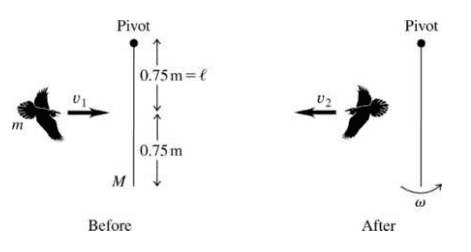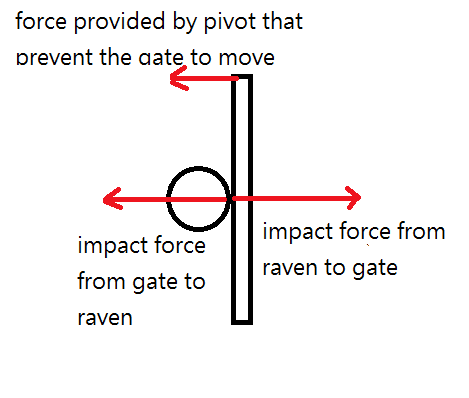# Linear and Angular Momentum on a wooden gate

## Homework Statement

A uniform, 4.5-kg, square, solid wooden gate 1.5 m on each side hangs vertically from a frictionless pivot at the center of its upper edge. A 1.1-kg raven flying horizontally at 5.0m/s flies into this door at its center and bounces back at 2.0m/s in the opposite direction. (a) What is the angular speed of the gate just after it is struck by the unfortunate raven? (b) During the collision, why is the angular momentum conserved, but not the linear momentum?

## The Attempt at a Solution

I think that if there is no external torque, angular momentum should be conserved. Since the weight of the gate is in the same line of the gate, there should not be any contribution to torque and therefore angular momentum should be conserved. But how about the linear momentum? In my opinion, all the force should be balanced so that the gate will not move downward. The should not be any external force, however the answer stated that linear momentum is not conserved, because there is an external force exerted by the pivot. But is the force should still be balanced, even it will be spinning?

Last edited:

Andrew Mason
Homework Helper
Welcome to PF!

Can you provide a diagram? Could you also provide the wording of the problem exactly as written? Thanks.

AM

I can provide a diagram from the solutionsorry for not double check it before I post the question. Already edited the exact wording for the problem.

Doc Al
Mentor
The should not be any external force, however the answer stated that linear momentum is not conserved, because there is an external force exerted by the pivot.
Why don't you think the pivot exerts an external force?

What direction of the external force will the pivot exert? is it upward? But the force should be balanced for the gate and pivot. Do I mixed up with something else?

Doc Al
Mentor
The "system" you should be considering is the gate + raven. The pivot is external to the system.

Ok, now i should choose gate and raven as a system to consider whether the momentum is conserved. I classified the weight as one of the external force exerted by the earth. And if the pivot is external to the system, what is the direction of the external force that pivot exerted on the gate?

Doc Al
Mentor
And if the pivot is external to the system, what is the direction of the external force that pivot exerted on the gate?
The force from the pivot is whatever it needs to be to prevent that part of the gate from moving. (I would not worry about the details.)

What you need to understand is that the pivot, assumed to be frictionless, can exert a force but not a torque on the gate.

So if the linear momentum is not conserved, the net external force from the pivot and weight should not be zero, is it because the gate will rotate?
Sorry for poor understandingharuspex
Homework Helper
Gold Member
So if the linear momentum is not conserved, the net external force from the pivot and weight should not be zero, is it because the gate will rotate?
Sorry for poor understandingAs Doc Al wrote, if not for the pivot the whole gate would have moved. Therefore the pivot provided a horizontal impulse on the gate, and linear momentum of gate+bird is not conserved. You could include the pivot in the system, but then something else has to be holding the pivot fixed in space.
The force of gravity was being countered by a vertical force from the pivot, and the horizontal impact does not immediately alter that, so there is no net vertical force, so no net vertical impulse.

For angular momentum, it is always important to specify the reference axis. Since there is a horizontal impulse from the pivot, it could also result in an angular impulse, changing angular momentum. To avoid this, you must choose the pivot itself as the reference axis. A force through the reference axis has no moment about the axis: moment = force x perpendicular distance, and that distance will be zero. While the gate is vertical, gravity also acts through the pivot. So angular momentum about the pivot is conserved during the instant of the collision.

Subsequent to the collision, as the gate swings from the vertical, gravity will exert a moment about the reference axis, so angular momentum about it will start to change.

This is what I come up witham I correct?

haruspex
Homework Helper
Gold Member
This is what I come up with View attachment 99291 am I correct?
Yes, that's the right diagram. Now you need to use conservation of angular momentum about the pivot. What is the raven's angular momentum about the pivot before impact?

So the two impact forces of raven and gate are not classified as external force because they are inside the system (gate + raven) And the only external force is the force provided by the pivot. So that linear momentum is not conserved. I think I mixed up the conservation of momentum by divide the system into the gate and raven, so the force of the gate is balanced, therefore linear momentum is conserved. Thank you.

(1.1)(5)(1.5/2)=(1.1)(-2)(1.5/2)+(4.5)(1.5^2)(angular speed^2)/3

haruspex
Homework Helper
Gold Member
(1.1)(5)(1.5/2)=(1.1)(-2)(1.5/2)+(4.5)(1.5^2)(angular speed^2)/3
Very nearly right. The angular speed should not be squared. (Check the dimensions.). You probably got mixed up with rotational KE, or maybe centripetal acceleration.

(1.1)(5)(1.5/2)=(1.1)(-2)(1.5/2)+(4.5)(1.5^2)(angular speed)/3 I don't remember that L=moment of inertia * angular velocity instead of square the angular velocity. Thank you very much.

haruspex
Homework Helper
Gold Member
(1.1)(5)(1.5/2)=(1.1)(-2)(1.5/2)+(4.5)(1.5^2)(angular speed)/3 I don't remember that L=moment of inertia * angular velocity instead of square the angular velocity. Thank you very much.
Ok, so what do you get for the angular velocity of the gate?

•Tissue
angular velocity of the gate is 1.71rad/s

haruspex